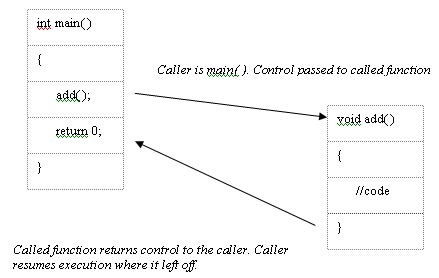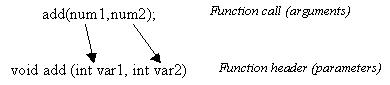Functions Intro

The following topics are covered in this section:

What is a function?

A function is a group of statements within a single unit. Basically, it is a block of executable statements grouped together which will be executed when the function is called. They are the building blocks of a program. You may wonder what’s the use of a function is? Or why not write the entire program within the main function itself?

Suppose in your program you have to repeat a certain set of statements at different instances, it wouldn’t be a good idea to keep repeating the same statements throughout the entire program. It will be ideal to place these statements within a function and use a single statement to call the function wherever needed (this approach makes it simpler to understand the program logic). Functions provide means for breaking down a large program into smaller modules. Using functions will also help in debugging programs (identifying and correcting errors).

The general syntax for a function declaration is:

return-data-type function-name (parameters);

The return data type specifies what sort of data the function will return to the calling process. For example if the return data type is ‘int’, then the function will return an integer value to the calling process. If the return data type is void, this means that the function does not return any value. What is a calling process? Every function has to be called by someone. A function will execute only when it is called. In C++, functions can be called by the main ( ) function or by some other function that you have defined. The main ( ) function is the part of your program that will execute and usually you will call the other functions from the main ( ) function.

You may wonder, the main ( ) itself is a function, then who calls the main ( ) function? The main ( ) function is the main part of your program and it is called by the operating system (since your OS will run the program).

There are three components for a function, namely:

1. Function Declaration or Prototyping
2. Function call and
3. Function definition.

Function Declaration (Prototyping):

Declaration means informing the compiler that a function, with this name and which looks like this, will appear later on in the program. It is a statement that specifies the function name, parameter data types and return data type. Function declaration is always done before (and outside) the main ( ) function block.
The syntax for declaring a function is:

return-data-type function-name (data-type-of-parameters);
Note that the function declaration should have a statement terminator.

Function Definition:

Once the compiler knows that you have declared a function, it then needs the definition for that function. The function definition contains the code to be executed whenever the function is called. The definition tells the compiler what it has to do when it encounters that function call.

The first line of the function definition is the same as the declaration and is called the ‘declarator’ (it is also called the function header). Don’t confuse declaration with declarator. The function header is very similar, in appearance, to the function declaration (or prototype) except that it does not have the statement terminator. The function header must agree with the prototype (i.e. it must have the same function name and the same data types of parameters in the same order and the also the same return data-type).

The general syntax of the three components of a function are as follows:

// Function declaration

return-data-type function-name (data type of parameter1,data type of parameter2) ;

int main ( )
{
function-name (arguments);
//Function call within main( )
}

// Function definition (outside main( ) )

return-data-type function-name (arguments) // Declarator/ Header
{
// Function Code or Body of function
}

Parameters and arguments will be dealt with later in this chapter. Let us consider an example to illustrate the three basic components of a function.

#include <iostream.h>
// Function Declaration
int main( )
{
// Function Call
return 0;
}

{
int num1,num2,sum;
// Body of the function
cout<< "Input the two numbers : ";
cin>>num1;
cin>>num2;
sum = num1 + num2;
cout<<"The sum is "<<sum;
}

As you can see, we have first declared that there is a function named ‘add’ with the return data type as ‘void’ and with no parameters. When ‘void’ is used as the return data type it means that the function will not return anything to the caller.

Remember: The compiler will always execute what you type in the main ( ) function. You can execute other functions by calling them from within the main ( ) function.

We call the function add( ) from within the main ( ) function. When the compiler encounters the function call statement, it recollects that you had already declared a function by the same name and argument type. So it goes on reading your program assuming that you have defined the function later in the program. In the definition we have written a set of statements to find the sum of two numbers. The compiler will now know what to execute when it encounters the add ( ) function. After displaying the result (due to the presence of ‘cout’ in the ‘add’ function), the program flow will go back to the statement following the function call in the caller. In this case the caller is main ( ) and the next line is

return 0;There are some finer details that you should note. When a function calls another function, the caller does not terminate. Instead it waits for the called function to return back control. The caller sleeps till it receives control again. There is some overhead involved in this process because the program needs to store extra information to know exactly where to return to. The program also needs to keep track of variables used in the calling function because once control is returned back to the caller, these variables can be used. This might seem confusing but just remember that extra operations (overhead) is involved in calling functions. You’ll understand this better once you learn recursion. Coming back to our example, the function declaration will tell the compiler that a function by that name is coming up later on in the program. There is a way to avoid writing the function declaration; i.e. there is no need to declare the function. In the above program the function definition comes after the function call. If you do not want to declare the function, the function definition must come before (precede) the function call.

Let us consider the same program to add two numbers using a function called add with no arguments.

Example 2:

// When function definition precedes function call
#include <iostream.h>
{
int num1,num2,sum;
cout<< "Input the two numbers : ";
cin>>num1;
cin>>num2;
sum = num1 + num2;
cout<<"The sum is "<<sum;
}

int main ( )
{
return 0;
}

As you can see, in the above program there is only one small change. The function add ( ) hasn’t been declared because it has been defined before the function call. The compiler will initially itself read the function definition and hence it knows two things before entering the main ( ) function – it knows that a function with the name ‘add’ will come up later in the program and it also knows what to do when it encounters the add ( ) function (because we’ve already defined the function).

Parameters and Arguments

Argument is a piece of data passed from the program to the function. Arguments allow a function to operate with different values. The variables used within the function to hold argument values are called parameters. The data type of parameters in the function declaration and data type of arguments in the function call should be the same.

Consider the following program to explain arguments. Again consider the same program to add two given numbers but using function arguments.

#include <iostream.h>
void add (int var1, int var2)
// Parameters var1 and var2
{
int var3;
var3 = var1 + var2;
cout<<"The sum of the two numbers is "<<var3;
}
int main( )
{
int num1, num2;
cout<< "Input the two numbers ";
cin>>num1;
cin>>num2;
//Arguments num1 and num2
return 0;
}

Two arguments have been passed to add ( ) from the main ( ) function. Observe the function ‘declarator/header’. The parameter specified is ‘int var1’ and ‘int var2’. This means that in the function named ‘add’, ‘var1’ and ‘var2’ are integers that are used within the body of the function. Within the function body we have declared a third integer called ‘var3’.
var1 and var2 are added and stored in var3 using the statement :

var3=var1 + var2;

Now the question might arise, what are the values for var1 and var2? In the main ( ) function the value for two integers ‘num1’ and ‘num2’ are obtained from the user. After obtaining the values, the following statement is encountered:

This statement is the function call. Two arguments namely ‘num1’ and ‘num2’ are passed through this function call. Since the function was declared and defined as having two parameters (and since the data types of the parameters and the arguments are the same), the program will execute the code of the add ( ) function with the value of ‘var1’ being that of ‘num1’ and value of ‘var2’ being equal to ‘num2’. If the data types or number of arguments passed do not match with the parameters, the compiler will produce an error message.

Effectively, var1=num1 and var2=num2. The body of the function ‘add’ is now executed with the values of ‘var1’ and ‘var2’. Thus the arguments are passed from the program (which is the main ( ) function) to the parameters of the function ‘add’.

The variables used to hold the argument values are known as parameters. The function declarator in the function definition specifies both data type and name of parameters. In our example, the parameters were ‘num1’ and ‘num2’. Their data types were int (integer).Remember: The values of ‘num1’ and ‘num2’ are passed to ‘var1’ and ‘var2’. The function is operating only on ‘var1’ and ‘var2’ (it does not operate on ‘num1’ and ‘num2’).

If we had declared the add ( ) function in the program, it would have been as follows: